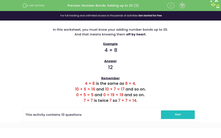# Use Simple Addition Skills Including Number Bonds to 20

In this worksheet, students will be tested on their knowledge of addition number bonds up to 20.Key stage:  KS 1

Curriculum topic:   Number: Addition and Subtraction

Curriculum subtopic:   Add and Subtract to 20

Difficulty level:#### Worksheet Overview

In this activity, there will be a mixture of questions. Some will be number bonds to 10 and 20 and some will be simple addition questions.

These penguins are going to remind us of our number bonds to 10:Remember that with our number bonds, the numbers can be swapped over.

For example, 2 + 8 go together to make 10 and so does 8 + 2.

Now, let's remind ourselves of the number bonds to 20And here are the rest:Remember that with our number bonds, the numbers can be swapped over.

For example, 7 + 13 go together to make 20 and so does 13 + 7.

Do your best to look through these and try to remember them.

Example 1

1 + ? = 10

We need to remember what number goes with 1 to make 10.

If we look back at our penguins, we can see that it is 9 that goes with 1 to make 10. So, our answer is 9.

Example 2

? + 12 = 20

Look back at the penguins and we can see that 8 goes with 12 to make 20. So, our answer is 8.

4 + 8 is the same as 8 + 4.

10 + 6 = 16 and 10 + 7 = 17 and so on.

0 + 5 = 5 and 0 + 19 = 19 and so on.

Are you feeling ready to have a go at some questions?### What is EdPlace?

We're your National Curriculum aligned online education content provider helping each child succeed in English, maths and science from year 1 to GCSE. With an EdPlace account you’ll be able to track and measure progress, helping each child achieve their best. We build confidence and attainment by personalising each child’s learning at a level that suits them.

Get started